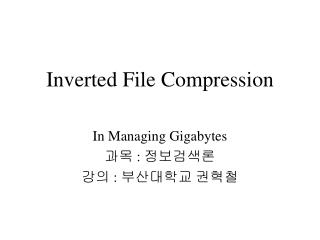DownloadDownload PresentationInverted File Compression

# Inverted File Compression

Télécharger la présentation## Inverted File Compression

- - - - - - - - - - - - - - - - - - - - - - - - - - - E N D - - - - - - - - - - - - - - - - - - - - - - - - - - -
##### Presentation Transcript

1. Inverted File Compression In Managing Gigabytes 과목 : 정보검색론 강의 : 부산대학교 권혁철

2. Inverted File Compression • Inverted file entry • <t; ft; [d1, d2, …, dft]> • t : term, ft : # of documents • dk : document no. where dk < dk+1 • < elephant; 8; [3, 5, 20, 21, 23, 76, 77, 78] > => < elephant; 8; [3, 2, 15, 1, 2, 53, 1, 1] > • gap = dk+1 -dk • Two compression classes • Global Methods V.S Local Methods

3. Summary of coding methods

4. Unarycode • Simple method • fixed representation of the positive integer • log N (bits) • Unary code • gap이 x일 때, x-1 bit의 1과 1bit의 0으로 표현 • lx = (x - 1) + 1, Pr[x] = 2-x • eg) x = 9 일 때, => 111111110

5.  code •  code • 1 + log x bit의 unary code와 log x bit의 binary code(x - 2log x)로 표현 • lx = 1 + log x + log x, Pr[x] = 1/2x2 • eg) x = 9 일 때, log x = 3, x - 2log x=1 => 1110001 • V = <1, 2, 4, 8, 16,…> or V = <1, 2, 2, 4, 4, 4, 8,…> or ….

6.  code •  code •  code와 표현 방법이 유사. • 1 + log x bit의 unary code대신에  code를 사용하고, log x bit의 binary code(x - 2log x)로 표현 • lx = 1 + 2log(1 + log x) + log x, Pr[x] = 1/2x(log x)2 • eg) x = 9 일 때, => 11000001

7. Global Bernoulli model • Pr[x] = (1-p)x-1p, p : gap x가 나타날 확률 • Golomb code • q + 1 bit의 unary code와 + log b or log b bit의 binary code • q = (x - 1) / b, r = x - q b - 1 • bA =log(2 - p) / - log(1 - p) 0.69(N n / f) • eg) b=3, r=0(0), 1(10), 2(11) b=6, r=0(00), 1(01), 2(100), 3(101), 4(110), 5(111) x=9이면, q = 2, r = 2 따라서, 11011

8. Global “observed frequency” model • Based on observed frequency of appear gap size • Use arithmetic or Huffman code • In theory • better compression method • In practice • slightly better than  and  code

9. Local Bernoulli model • The frequency of term t, ft , is known • Bernoulli model on each individual inverted file entry can be used • Very common words are encoded with b=1. • Tantamount bitvector • thus, inverted file can never worse than bitvector. • Necessary to store the parameter ft • b can be used during decoding

10. (a) (b) (c) Word position in Bible : (a)bridegroom; (b)Jezebel; (c) twelfth Skewed Bernoulli model • Bernoulli model의 vector VG = <b, b, b, …> • VT = <b, 2b, 4b, 2ib, …> • slightly worse than the Golomb code

11. Local hyperbolic model • Pr[x] =  / x, x = 1, 2, …, m •  = 1 / (loge(m+1)+0.5772) • m is largest gap • Better performance • more complex to implement • requires the use of arithmetic coding

12. Local “observed frequency” model • The ultimate in local modeling • batched frequency • request more memory space • best compression method

13. Performance of Index Compression Methods Method Bits per pointer Bible GNUbib Comact TREC Global methods Unary 264 920 490 1719 Binary 15.00 16.00 18.00 20.00 Bernoulli 9.67 11.65 10.58 12.61  6.55 5.69 4.48 6.43  6.26 5.08 4.36 6.19 Observed frequency 5.92 4.83 4.21 5.83 Local methods Bernoulli 6.13 6.17 5.40 5.73 Hyperbolic 5.77 5.17 4.65 5.74 Skewed Bernoulli 5.68 4.71 4.24 5.28 Batched frequency 5.61 4.65 4.03 5.27

14. Compression of bitmaps • Bitmaps : Hierarchical bitvetor compression기법으로 압축 (a) original bitvector (b) hierarchical structure (c) flattened tree as a string of bits